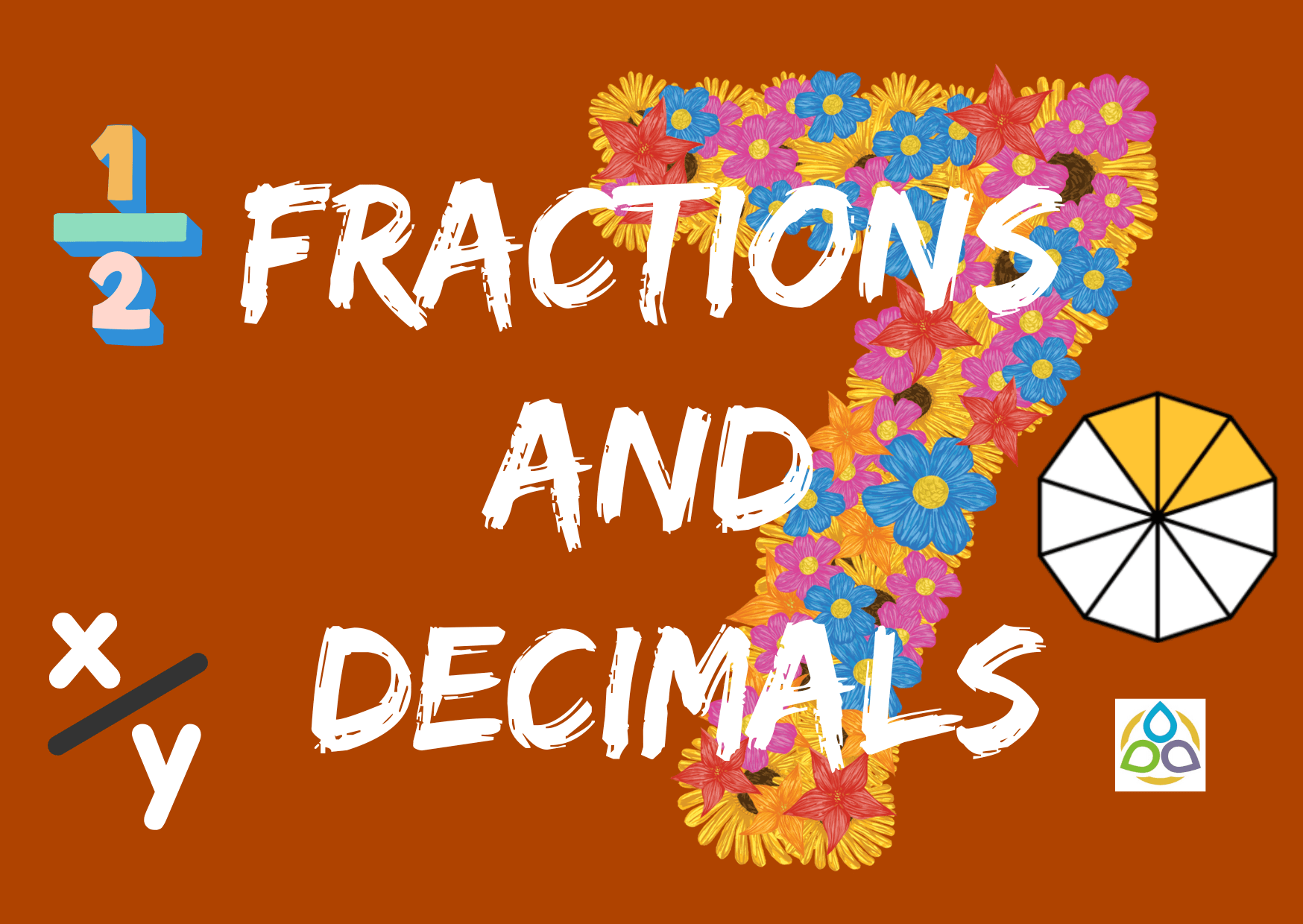# Fractions and Decimals

78. Raju scored 9 marks in math test. If the maximum marks of the test are 25, how is Raju’s score represented as a fraction?

(A) 1/25

(B) 16/25

(C) 9/25

(D) 25/25

76. Two fractions are equivalent if their cross-multiplications are

(A) 0

(B) 1

(C) Equal

(D) Not equal

75. Three people share an apple equally. What is the share of each?

(A) 1

(B) One-third

(C) Half

(D) One-fourth

74. If the numerator and denominator of a fraction are equal, then the fraction:

(A) is equal to 1

(B) is less than 1

(C) is equal to 0

(D) is greater than 1

73. If the numerator and denominator of a fraction are equal, then the fraction:

(A) is equal to 1

(B) is less than 1

(C) is equal to 0

(D) is greater than 1

72. Which of the following is a proper fraction?

(A) 4/3

(B) 3/4

(C) 13/4

(D) 21/5

71. What is the whole number present in the fraction 27/5?

(A) 4

(B) 5

(C) 6

(D) 5½

70. While converting a fraction to its simplest form, we divide both the numerator and denominator by their

(A) LCM

(B) HCF

(C) Product

(D) Difference

69. Which of the following is the difference between 7/8 and 3/8?

(A) 4/5

(B) 1/2

(C) 3/2

(D) 10/5

68. A fraction equivalent to 45/105 is

(A) 6/14

(B) 4/7

(C) 5/7

(D) 7/5

67. Which of the following is a fraction equivalent of 2/3?

(A) 4/5

(B) 8/6

(C) 10/25

(D) 10/15

66. What type of fraction is the sum (3/4) + (2/4)?

(A) Proper fraction

(B) Improper fraction

(C) Mixed fraction

(D) Unit fraction

65. A fraction equivalent to 3/5 is

(A) (3 + 2)/(5 + 2)

(B) (3 – 2)/(5 – 2)

(C) (3 × 2)/(5 × 2)

(D) (3 ÷ 3)/(5 ÷ 5)

64. Which of the following pairs of fractions are not equivalent?

(A) 1/2, 2/3

(B) 4/5, 12/15

(C) 6/7, 12/14

(D) 4/9, 36/81

63. Which among the following is the correct value of 1 – (1/2)?

(A) 1/2

(B) 1

(C) – 1/2

(D) 1/4